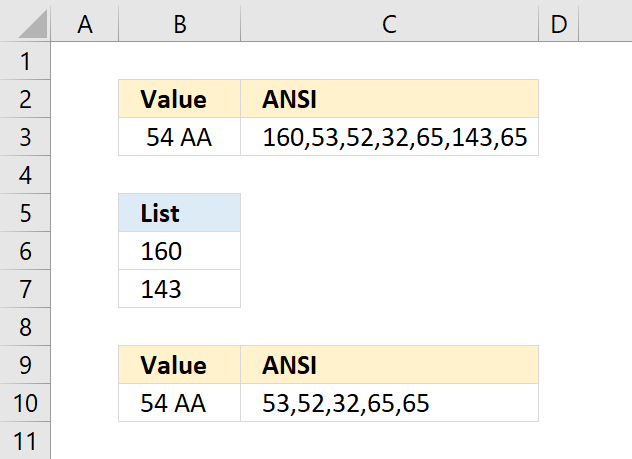Author: Oscar Cronquist Article last updated on November 15, 2018Cell B3 contains a few odd characters and the formula in C3 shows the ANSI equivalent of each character in B3. If you are interested in how I built the formula, read this: How to identify characters in a cell value

For example, 160 is an HTML space character that the TRIM and CLEAN function can't remove. You can remove a single character using the SUBSTITUTE function, however, if your cell contains multiple unwanted characters the following formula will remove specific characters.

Array formula in cell B10:

=TEXTJOIN(, TRUE, IF(COUNTIF(B6:B7, CODE(MID(B3, ROW(\$A\$1:INDEX(\$A\$1:\$A\$1000, LEN(B3))), 1))), "", MID(B3, ROW(\$A\$1:INDEX(\$A\$1:\$A\$1000, LEN(B3))), 1)))

The characters I want to remove are in B6:B7 in ANSI code format. The formula in cell C10 shows that 160 and 143 are now gone in cell B10.

### Explaining formula in cell B10

The INDEX function and the LEN function allows you to create a cell reference containing as many rows as there are characters in cell B3.

\$A\$1:INDEX(\$A\$1:\$A\$1000, LEN(B3))

becomes

\$A\$1:INDEX(\$A\$1:\$A\$1000, 7) and returns \$A\$1:\$A\$7.

The ROW function then creates an array from 1 to the number of characters in cell C3.

ROW(\$A\$1:INDEX(\$A\$1:\$A\$1000, LEN(B3)))

becomes

ROW(\$A\$1:\$A\$7) and returns {1;2;3;4;5;6;7}

Now it is time for the MID function to split each character in cell C3 to an array.

MID(B3, ROW(\$A\$1:INDEX(\$A\$1:\$A\$1000, LEN(B3))), 1)

becomes

MID(B3, {1;2;3;4;5;6;7}) and returns {" ";"5";"4";" ";"A";"";"A"}

The CODE function allows you to convert a character to ANSI code (PC). We are using an array so the function will convert all characters at the same time.

CODE(MID(B3, ROW(\$A\$1:INDEX(\$A\$1:\$A\$1000, LEN(B3))), 1))

becomes

CODE({" ";"5";"4";" ";"A";"";"A"}) and returns {160;53;52;32;65;143;65}

The COUNTIF function counts how many times 160 and 143 are found in the array.

COUNTIF(B6:B7, CODE(MID(B3, ROW(\$A\$1:INDEX(\$A\$1:\$A\$1000, LEN(B3))), 1)))

becomes

COUNTIF({160;143}, {160;53;52;32;65;143;65}) and returns {1;0;0;0;0;1;0}

The IF function checks the logical expression and returns a blank value if TRUE and the charcater if FALSE. The IF function evaluates TRUE as 1 and FALSE as 0 (zero).

IF(COUNTIF(B6:B7, CODE(MID(B3, ROW(\$A\$1:INDEX(\$A\$1:\$A\$1000, LEN(B3))), 1))), "", MID(B3, ROW(\$A\$1:INDEX(\$A\$1:\$A\$1000, LEN(B3))), 1))

becomes

IF({1;0;0;0;0;1;0},"",{" ";"5";"4";" ";"A";"";"A"}) and returns {"";"5";"4";" ";"A";"";"A"}.

Lastly, the TEXTJOIN function concatenates the remaining characters in the array ignoring blank values.

TEXTJOIN(, TRUE, IF(COUNTIF(B6:B7, CODE(MID(B3, ROW(\$A\$1:INDEX(\$A\$1:\$A\$1000, LEN(B3))), 1))), "", MID(B3, ROW(\$A\$1:INDEX(\$A\$1:\$A\$1000, LEN(B3))), 1)))

becomes

TEXTJOIN(, TRUE, {"";"5";"4";" ";"A";"";"A"}) and returns 54 AA in cell B10.